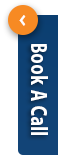﻿ rf filter, attenuator, power splitter, rf cable, connector and related emc components from ucon(shenzhen) technology limited companyBook call on your favorite timeAmazon Services

# ucon(shenzhen) technology limited company

Xin Yongsheng Building 702, Songgang Avenue 72,Bao'an District,Shenzhen,China,China

# 产品: 25

### 2 way power splitter

The UC-S*2 GPS Splitter is a one-input, two-output GPS device. This product typically finds application where an input from an active GPS roof antenna

### 2-way power Splitter

A power divider is a device that divides one channel of input signal energy into multiple channels to output equal or unequal energy. In turn, it can

### 2-way power Splitter

A power divider is a device that divides one channel of input signal energy into multiple channels to output equal or unequal energy. In turn, it can

### 2-Way Power Splitter

A power divider is a device that divides one channel of input signal energy into multiple channels to output equal or unequal energy.

### 4-Way Power Splitter

A power divider is a device that divides one channel of input signal energy into multiple channels to output equal or unequal energy. In turn, it can

### 4-Way Power Splitter

A power divider is a device that divides one channel of input signal energy into multiple channels to output equal or unequal energy. In turn, it can

### 3-Way Power Splitter

A power divider is a device that divides one channel of input signal energy into multiple channels to output equal or unequal energy. In turn, it can

### 12 way power splitter

A splitter is a device that divides one channel of input signal energy into multiple channels to output equal or unequal energy. In turn, it can synth

### 8 way Power splitter

A splitter is a device that divides one channel of input signal energy into multiple channels to output equal or unequal energy. In turn, it can synth

### 8 way power splitter

A splitter is a device that divides one channel of input signal energy into multiple channels to output equal or unequal energy. In turn, it can synth

### 16-Way power splitter

A power divider is a device that divides one channel of input signal energy into multiple channels to output equal or unequal energy. In turn, it can

### 4-way active splitter

A power divider is a device that divides one channel of input signal energy into multiple channels to output equal or unequal energy. In turn, it can

### 2-Way power splitter

A power divider is a device that divides one channel of input signal energy into multiple channels to output equal or unequal energy. In turn, it ca

### 2-Way power splitter

A power divider is a device that divides one channel of input signal energy into multiple channels to output equal or unequal energy. In turn, it can

### 2-way power Splitter

A power splitter is a device that divides one channel of input signal energy into multiple channels to output equal or unequal energy. In turn, it can

### 2-way power Splitter

A power splitter is a device that divides one channel of input signal energy into multiple channels to output equal or unequal energy. In turn, it can

### 3-Way power splitter

A power divider is a device that divides one channel of input signal energy into multiple channels to output equal or unequal energy. In turn, it can

### 3-Way power splitter

A power divider is a device that divides one channel of input signal energy into multiple channels to output equal or unequal energy. In turn, it can

### 3-Way power splitter

A power splitter is a device that divides one channel of input signal energy into multiple channels to output equal or unequal energy. In turn, it can

### 4-way power splitter

A power splitter is a device that divides one channel of input signal energy into multiple channels to output equal or unequal energy. In turn, it can

### 5-way power splitter

A power splitter is a device that divides one channel of input signal energy into multiple channels to output equal or unequal energy. In turn, it can

### 6 way power splitter

A power splitter is a device that divides one channel of input signal energy into multiple channels to output equal or unequal energy. In turn, it can

### 8-way power splitter

A power splitter is a device that divides one channel of input signal energy into multiple channels to output equal or unequal energy. In turn, it can

### 10-way power splitter

A power splitter is a device that divides one channel of input signal energy into multiple channels to output equal or unequal energy. In turn, it can

### 16-way power splitter

A power splitter is a device that divides one channel of input signal energy into multiple channels to output equal or unequal energy. In turn, it can

## 联系我们

联系人: Amelia Guo

公司名称:ucon(shenzhen) technology limited company

办公地址:Xin Yongsheng Building 702, Songgang Avenue 72,Bao'an District,Shenzhen,China,

电话号码:

查看電話號碼

移动电话:

查看手機號碼

### 至,

Amelia Guo < ucon(shenzhen) technology limited company >
﻿